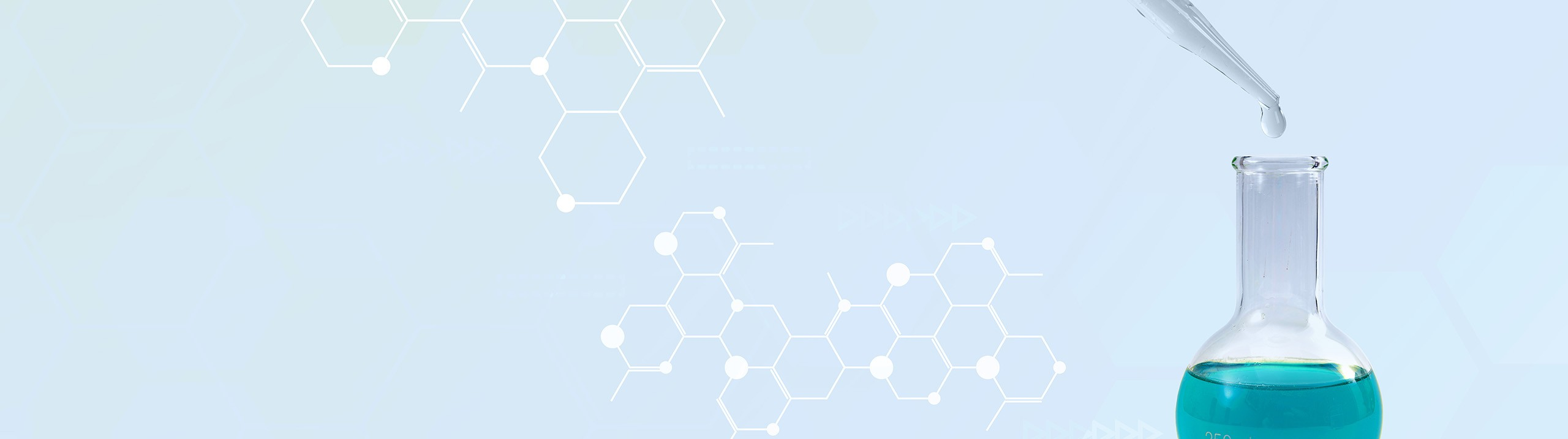## Analytical Chemistry

Learn the toughest concepts covered in your Analytical Chemistry class with step-by-step video tutorials and practice problems.

6. Chemical Equilibrium

# The Equilibrium State

Under an equilibrium state the rates of the reverse and forward reaction are equal.

The Equilibrium State
1
concept

## The Equilibrium State6m
Play a video:
when it comes to chemical reactions, were gonna say that many of them never go to completion. This means that react ints do not completely convert into products and as a result reacting concentrations do not go down to zero. We're going to say that these types of chemical reactions reach a chemical equilibrium in which the forward and reverse reactions are happening. What this means is, for example, we have three moles of H two gas reacting with one mole of N two gas to produce two moles of ammonia gasses product. What happens is initially all we have are reacting. It's over time those reactions will break down in order to recombine to form this product. We're gonna say moving in this forward direction to make product we have associated with it, our rate constant in the forward direction. When enough time has passed, the products have built up to a sufficient number, it will begin to break down and move in the reverse direction to reform our reactant that has associated with it, the rate of rate constant for the reverse direction. Now, when it comes to the rate constants, they're tied to rate here in our forward rate and our reverse rate, we're gonna say initially again, we only have react ints. So we're gonna start out with our forward rate in which reactant break down to form products. Realize here that over time this forward rate will decrease. That's because we're having less and less reactant that can break down. So the forward rate is slowing down at the same time, we have no reverse rate initially because there's no products, but once our products reach a sufficient amount, it will begin to break down and reform our reactant. When that starts to happen, we start to get a reverse rate over time both of these rates will reach a number in which they are equal when the rates become equal in both the forward and reverse direction. That is when equilibrium is established. Now with equilibrium we have our capital K variable here, which is our equilibrium constant. The equilibrium constant equals your rate constant in the four direction, divided by your rate constant in the reverse direction. It is also equal to products. Overreact ints Now remember in the latter part of chemistry, Jen came when you guys took it. Remember what associated with our equilibrium constant is our equilibrium expression here. We ignore solids and liquids we're gonna have here. NH three the coefficient becomes the power so that's squared Divided by age two Cubed Times and two. So this would represent our equilibrium expression or equilibrium equation. Now, if we had actual values for the products and reactions, we could plug them in and find a numerical value for our equilibrium constant. K. By knowing what that value is, we can determine which side of the equation is favored. Um Is the product side favored meaning that our reaction move more in the forward direction to make more products or is it favored in the reverse direction? Now, if we're gonna talk about that we have to take a look here at these three images. Now in the first image, realize here we have our reactant a Our product B. We start out with some reactant initially no products initially. Over time our reactant break down to form products. Realize here because of that our reactions over time are decreasing while our product is increasing Around the five minute mark. We can see that they're amounts reach a plateau where they stop changing it. Is that that exact moment? At five minutes where equilibrium is established here again, remember K. Equals products overreacting. So that's B over A. at Equilibrium. It looks like B is equal to around eight A. is equal to round one. So Kay here is eight, we're gonna say here when K is more than one or greater than one. That means that the ford direction is favored and products are favored because if your favorite in the forward direction, that means you're moving the forward direction more. So to help make more products in this one here we can see that our reactions are still decreasing. Products are still um reactions are decreasing. Products are increasing. But at the end, when equilibrium is established, we still have more reacting than we do product. So he will say this is around six. It looks like and then be here looks like it's around just over four. So in this situation you can see that your K. is less than one and that means that the reverse direction is more favored and that means your reactant, our favorite. So again, the magnitude for K. Weather, K is equal to or greater than greater than one. It means that products are favored if it's less than one, that means reactions are favored in this last image at five minutes, they both meet at around five. So Kay here equals one, which means that we are at ideal equilibrium. So when K equals one, that's more ideal equilibrium. So it's equal to one. So no direction is favored and products and reactions are both favored. So just realize that when it comes to the equilibrium of a chemical reaction, it's moving both in the forward and reverse direction. Because the rate of the forward direction, the rate of the reverse reaction get to a value that's equal after X. Amount of time. At that moment chemical equilibrium can be established um As we go deeper and deeper into chemical equilibrium, we'll talk about additional topics um such as equilibrium concentrations of reactions and products at the end. Ice charts of course as well as other important topics. But fundamentally remember that equilibrium cannot be established until the rate of the forward and the rate of adverse reactions get to an equal value. Yeah

Equilibrium is reached when the rates of the forward and reverse reaction are equal.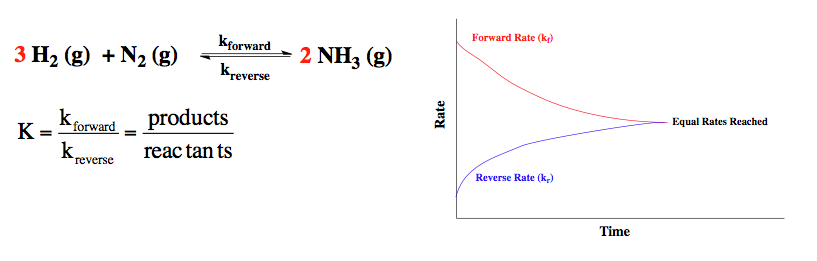The magnitude of K determines which direction is favored at equilibrium. If K is greater than 1 then products are favored over reactants.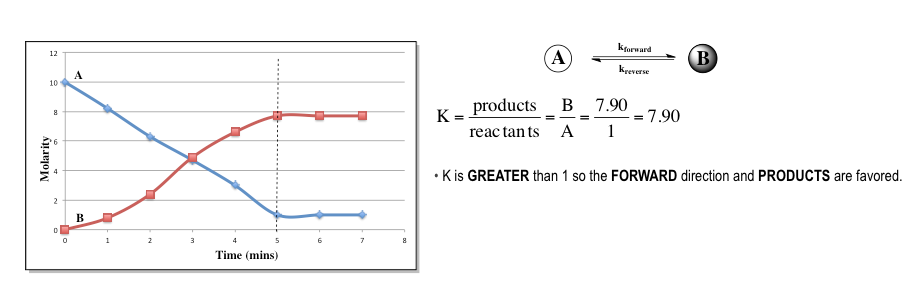If K is less than 1 then reactants are favored over products.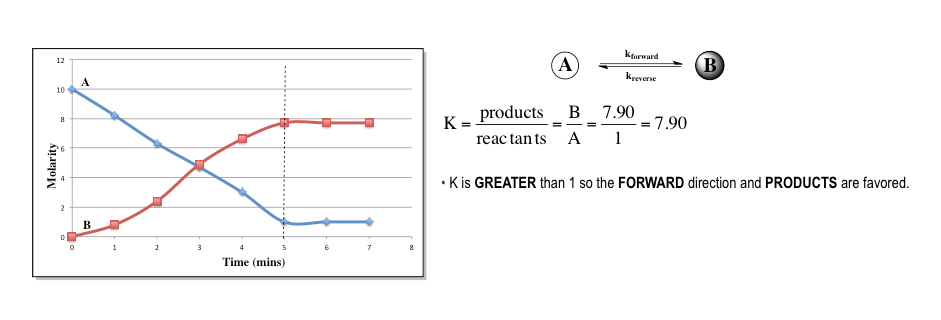If K is equal to 1 then both reactants and products are favored.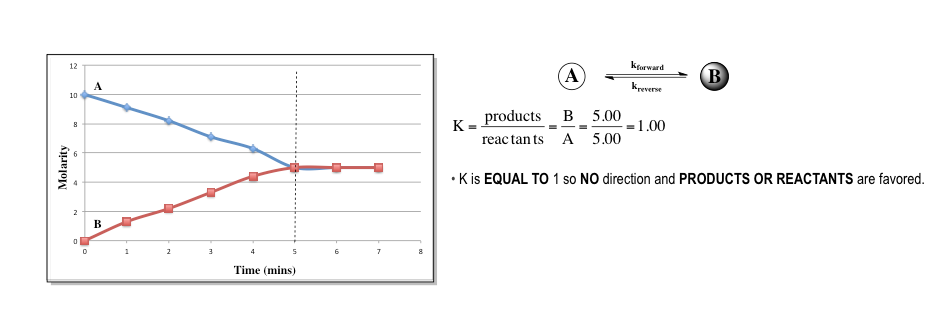Equilibrium State Calculations
2
example

## Equilibrium State Calculations 13m
Play a video:
So here we're told for the following chemical reaction, nitrogen gas plus, oxygen gas produces two moles of nitrogen monoxide gas. We're told that our equilibrium constant K. C is 3.7 times 10 to the negative five. Our rate constant for the four directions, 2.5 times 10 to the negative three. And our rate constant for the reverse reaction is 67.57. Now they're saying addition of a catalyst increases the ford rate constant um to 1.8 times 10 to the negative one. What is the new reverse rate constant after the addition of the catalyst? So remember the equation is our equilibrium constant. K. C equals our rate constant in the forward direction, divided by a rate constant in the reverse direction. Now, when it comes to our rate constants, they can be affected by a few factors, they can be affected by the addition of a catalyst. They can be affected if we increase or decrease are reacting concentration, they can be affected by increasing the surface area of our reactant since they can also be affected by a change in temperature. The addition of a catalyst here is the direct factor in changing my ford rate constant from 2.5 times 10 times 10 to negative 3 to 1.8 times 10 to the negative one. Now, when it comes to our equilibrium constant though. So capital K, the only factor that can affect its value, make it different would be temperature because we never mentioned a change in temperature here. That means that our equilibrium constant K C will remain the same value. So it's still going to be 3.7 times 10 to the negative five even after the addition of a catalyst here, the rate constant in the four direction we're told is 1.8 times 10 to the negative one. And all we're doing here is solving for rate constant in the reverse direction. So we're just solving for K. Are there all we have to do is isolate KR. So multiply both sides by KR So K. R. Times my equilibrium constant equals The rate constant in the four direction. And now we just need to divide both sides here by our equilibrium constant to isolate the rate constant in the reverse direction. Yeah, So when I do that now KR from my reverse direction equals 4.86 times 10 to the three as my final answer. So just remember the factors affecting the rate constant. Um are these four? Whereas to affect our equilibrium constant, all that we can do is affect temperature and that will cause a change in my equilibrium constant. We'll talk in greater detail on if this increases or decreases in my equilibrium constant. When we discuss the settlers principle. Also, what you should keep in mind in terms of this question is the addition of a catalyst. Not only increased the rate of the forward reaction, but it also affects the reverse reaction. So, catalyst affects both the reverse and forward directions in a chemical reaction. Now that you've seen this, go onto the next video and take a look at example, too. If you want, you can attempt it on your own. But again, if you get stuck, don't worry, just come back and see how I approach example, too.
3
example

## Equilibrium State Calculations 12m
Play a video:
Yeah, consider the following reactions in 25 degrees Celsius. So in reaction one, we have two moles of N. O. Decomposing to produce N two gas and 02 gas here, it has a K value of one times 10 to the 30 reaction to. We have two moles of water breaking down to give us two moles of hydrogen gas and one mole of oxygen gas. It has a K value of five times 10 to the negative 82. And then finally we have two moles of carbon monoxide plus one mole of oxygen gas combining to give us two moles of carbon dioxide gas. Here it has a K value of three times 10 to the negative 92. The positive 91. Now here it says which compound is most likely to disassociate and give 02 gas as at 25°C. Alright, so they're saying here that we have a compound, it's going to dissociate or break up To produce 02. So, 02 has to be a product. When we look at our three equations automatically. The third equation is out Because in this 102 is not a product, it's a reactant. And again, we're looking for a compound to break apart to produce 02 as a product. Alright, so now we have the answer is looking at either reaction one to reaction to. Now we want to create 02 as a product And realize here that our equilibrium constant or capital K. looks at the magnitude of our equation basically, the larger your K value is the more products you can produce here in reaction one, this equilibrium value, equilibrium constant value is really large, which means that we produce a great amount of product so we'll produce a lot of 02 gas. And what helps to produce that 02 gas, nitrogen monoxide breaking up helps to produce that 02 gas. So the answer here would be option B reaction to doesn't work because here it's equilibrium constant is much smaller than one, which means that reactant are much more greatly favored. So we're not going to produce very much product at all. So very little of 02 would be produced. So that's why H 20 gas could not be an answer. And remember this all hinges on the fact that K. Your equilibrium constant equals products. Overreacting. If you're K is larger than one, then product would have to be a much larger number than reactant. That's what makes reaction one the best choice. And that's why Eno gas is the correct compound, which will break up to produce the most amount of 02. I do
4
Problem

Write the equilibrium expression for the following reaction.

4 NH(g) + 3 O(g) ⇌  2 N(g) + 6 H2O (l)

5
example

## Equilibrium State Calculations 23m
Play a video:
when reaction one and two below are added together. The result is reaction three were asked to find the equilibrium constant for reaction three, which is K three. Now, the way we approach this question is the way we would approach a question if we're dealing with Hess's Law. Our goal is to isolate this equation here. And the way we isolate that equation is by manipulating the other equations that have known equilibrium constant values. Alright, so we're gonna go one by one and see what we find each one of these compounds. So here we need one mole of nitrous acid as a reactant. If we look up above that, the reactions one and two here, we have one mole of nitrous acid as a reactant. It matches exactly with the one mole that I need here. That tells me that I cannot manipulate reaction one at all. So I leave it as is next, I need one mole of hydroxide ion as reactant. And here it goes here again, it matches perfectly, which means I don't do anything to this equation. I don't manipulate it at all. And then we can see here that my one mole of an L two minus as a product that I need is right here and my one mole of water as a liquid as a product. I need one mole here, I have two moles of it as products. But then I also have one mole of it over here is reacting. It's what's going to happen is that this one's gonna completely cancel out with one from here, Leaving me with one left, everything that hasn't canceled. That would come down. So this would come down to give me this. This also cancels out with this. This would come down to give me this. This would come down to give me this and this would come down to give me this. I've canceled out everything that I can cancel out. And I've isolated the equation that I need always say at this point is to find K three, we would say K three equals K one times K two. So we'd multiply 4.50 times 10 to the negative, four times 1.0 times 10 to the 14 when we do that. It gives me a K three value of 4.50 times 10 to the 10. This one was pretty simple. We didn't have to manipulate the equations at all. All we had to do was cancel out intermediates which are compounds that look alike except one is a reacting, one is a product and then just multiply your equilibrium constants to find the missing one. In the bottom example, we will have to do some types of manipulations to equations to get the desired final equation. In that, in those cases we'll see what happens to our equilibrium constants when we manipulate the equations as a whole. So guys keep in mind the simple things that we did here as we move on to the next example in the next video. Okay
6
example

## Equilibrium State Calculations 36m
Play a video: